Search IntMath
Close

450+ Math Lessons written by Math Professors and Teachers

5 Million+ Students Helped Each Year

1200+ Articles Written by Math Educators and Enthusiasts

Simplifying and Teaching Math for Over 23 Years

# IntMath Newsletter: Interactive 3D graph, Polymath

By Murray Bourne, 19 Apr 2016

19 Apr 2016

1. New 3D interactive graph
2. Over 70 interactives
3. Roller door problem and Polymath software
4. Math in the news
5. Math puzzles
6. Math movie: Math symbols in ancient rock art?
7. Final thought: Mathematical habits of mind

## 1. New 3D interactive graph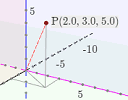I recently added a simple 3D interactive graph which allows you to explore the x-, y- and z-axis system. Here's some background (which includes a discussion about the "correct" orientation for the axes in a 3D graph): New 3D interactive graph

## 2. Now over 70 interactives!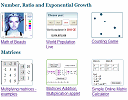There are now over 70 interactive math applets (most of them mobile-friendly) on IntMath. I recently updated the full list, which you can find here: Math Interactive Applets

## 3. Roller door problem and Polymath software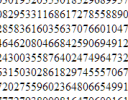Here's a numerical solution to an earlier problem we discussed involving the height of a roller door given the number of turns the door makes. Roller door problem and Polymath software

## 4. Math in the News

### (a) Andrew Wiles receives Abel Award for solving Fermat's Last TheoremSir Andrew Wiles solved Fermat's Last Theorem in 1994. He proved there are no integer solutions for n ≥ 3 in the equation xn + yn = zn. Wiles was recently awarded the Abel Prize "for his stunning proof of Fermat’s Last Theorem by way of the modularity conjecture for semistable elliptic curves, opening a new era in number theory".

His prize money is equivalent to \$850,000 (USD).

Last year's joint winners for the Abel Prize were John F. Nash Jr. (who was the subject of the movie A Beautiful Mind) & Louis Nirenberg.

### (b) Math Awareness Month: Making PredictionsApril is Math Awareness Month, and the theme this year is "Making Predictions". It's a good theme, and one that we should encourage more in mathematics courses. Making predictions requires mathematical modelling which is an incredibly important concept, but is rarely taught in schools.

The articles on the past, present and future of prediction and the central position of mathematics and statistics are quite interesting.

## 5. Math puzzles

The puzzle in the last IntMath Newsletter asked about a journey by two girls involving a bicycle and/or walking.

Correct answers with sufficient explanation were given by: Chris and Hamid. There was certainly an interesting assortment of solutions for this one!

### New math puzzle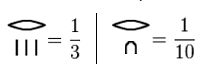The Egyptians used unit fractions, where the numerator was always 1.

They would need to write their ratios as the sum (not difference) of unique unit fractions, like this: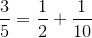Using this system, how would they have written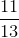?

You can leave your responses here.

## 6. Math movie: Geometric shapes in ancient rock art paintings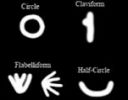Ancient rock art may give clues to the origin of written symbols, including early math notation. Geometric shapes in ancient rock art paintings

## 7. Final thought: Mathematical habits of mind

In the article Mathematical Habits of Mind, Cindy Bryant discusses the importance of developing sound strategies for solving math problems. Expert problem solvers don't panic when they come across an unfamiliar problem. They will use one or more of the following strategies (based on Polya''s four-step problem solving process) until something "clicks":

Draw a picture
Find a pattern
Make a list
Make a table
Solve a simpler problem
Guess and check
Act out the problem
Work backwards
Write an equation

Until next time, enjoy whatever you learn.

1. cccccttttt says:

Subtract 1/2 from 11/13.
From that subtract 1/3.
This last difference is 1/78.

(1/2)+(1/3)+(1/78)= 11/13

No wonder only priests and builders used fractions.

-----------------

Well done 3D applet.

Far better illusion than my hand drawings.

You may eventually offer an option

ct

2. Mike Thayer says:

Good problem!

11/13 = 1/2 + 1/3 + 1/78

3. Nour Hlwani says:

We know that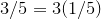And that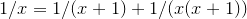So we conclude that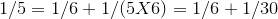And finally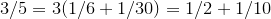Using the same technique for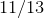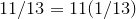And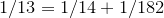Then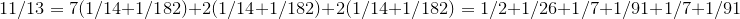We still need to clear the duplicates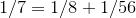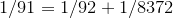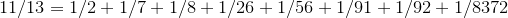4. Don Miller says:

1/2 + 1/3 = .833333 close to 11/13

let x= 11/13 - .833333, and y =1/x

By searching, y=78. So 1/2 +1/3 + 1/78 = 11/13

5. Lidia Carro says:

1/2 + 1/3 + 1/78 = 11/13

6. Rika says:

It is more than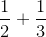,this minus and get the answer: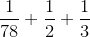7. Rika says:

Hi Murray
Hope you are well. I am still so thankful to you for your help with maths on internet 🙂
Regards
Rika

8. Murray says:

I'm well, Rika, and you're welcome!

9. Ben Murray says:

The unit fractions, in decreasing order of size, are 1/2, 1/3, 1/4, 1/5 and so on.

11/13, the fraction that we need to convert to unit fractions, Egyption-style, is nearly one. Definitely bigger than 1/2, so let's subtract 1/2 and see what we're left with:

11/13 - 1/2 = 22/26 - 13/26 = 9/26.

So we know that 11/13 = 1/2 + 9/26. We're making progress, but there's still more work to do.

9/26 is just a tiny bit bigger than 1/3, so let's take that away and see what remains:

9/26 - 1/3 = 27/78 - 26/78 = 1/78. Which, happily enough, is also a unit fraction.

Putting it all together, we can see that:

11/13 = 1/2 + 1/3 + 1/78. Numbers that the Eqyptians can relate to.

10. karam says:

So thank you for this wonderful effort
11/13 = 1/2 + 1/4 + 1/13 + 1 /52

11. Kjell O. Foss says:

11/13 = 1/2 + 1/4 + 1/13 + 1/52

12. george says:

1/2 + 1/3 + 1/26 = 11/13

13. Thomas A Buckley says:

11/13
= 22/26
= 13/26 + 9/26
= 1/2 + 18/52
= 1/2 + 13/52 +5/52
= 1/2 +1/4 + 4/52 +1/52
= 1/2 + 1/4 + 1/13 + 1/52
=
............
I I + IIII + n + III + n+n+n+n+n + II

Graphic limited ! Bah

14. Thomas A Buckley says:

My graphic looked OK in preview comment,
but lost numerator symbols when submitted.
So for clarity I've separated my 4 fractions, omitting the numerator symbol.

II, IIII, n+III, n+n+n+n+n+II
Furthermore the +'s may be omitted, if the numerator symbols are assumed to be added.
Yielding,
II, IIII, nIII, nnnnnII

15. Jess Grindlay says:

I split it into 3, and I got:
1/3 + 1/2 + 1/78

I times it through by 3, so I could split it into 1 third and then got a half from that and realised the remainder was only one.

### Comment Preview

HTML: You can use simple tags like <b>, <a href="...">, etc.

To enter math, you can can either:

1. Use simple calculator-like input in the following format (surround your math in backticks, or qq on tablet or phone):
a^2 = sqrt(b^2 + c^2)
(See more on ASCIIMath syntax); or
2. Use simple LaTeX in the following format. Surround your math with $$ and $$.
$$\int g dx = \sqrt{\frac{a}{b}}$$
(This is standard simple LaTeX.)

NOTE: You can mix both types of math entry in your comment.